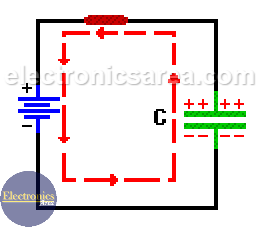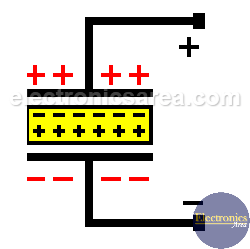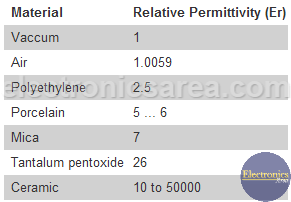# Capacitor and Direct Current

The capacitor is manufactured in many shapes and materials, but no matter how it has been constructed, it is always a device with two plates separated by an insulating material. If a battery is connected to a capacitor, it will allows no direct current (DC) to flow through.

If a not charged capacitor is connected across the terminals of a battery, a transient current flows as the capacitor plates charge up.

Current flows from the battery terminals to the capacitor plates.

• The positive battery terminal, attracts electrons from the upper capacitor plate. The plate is positively charged.
• The negative battery terminal, fill with electrons the bottom capacitor plate. The plate is negatively charged.

See the flow of electrons charging the capacitor plates in the diagram. The flow of electrons decreases slowly and finally stops. At this time the capacitor behaves like an open circuit for DC.

The current that is discussed in the preceding paragraphs is a current that varies over time, the current starts from a maximum value and decreases to 0 amps, when there is no current flowing. This happens in a very short period of time and is called “transient current”.The amount of charge a capacitor can store, is called “capacitance”. The capacitance value depends on the physical characteristics of the capacitor.

• The greater the area of the plates, the higher the capacitance.
• The smaller the gap between plates, the higher the capacitance.
• The type of dielectric used between the plates affects the capacitance value.The dielectric aims to increase the capacitance value of the capacitor. When a dielectric is used, it acquires a charge opposite to the charge on the plates, reducing the net charge of the device, allowing the arrival of more charge to the plates.

## Permittivity

Different materials are used as dielectrics, with different degrees of permittivity (Different degrees of ability to establish an electric field).The higher the permittivity, the higher the capacity the dielectric allows to obtain. Capacity is calculated using the formula: C = (Er x A)/d, where:

• C = capacity.
• Er = permittivity.
• A = plates’s area.
• D = distance between plates.

The unit of measurement of the capacitor is the Farad, but this unit is big and is more common use:

Look at: Definition of common units.

The main electrical characteristics of a capacitor are its capacity and maximum voltage between plates. There are two types of capacitors:

• Fixed Capacitors: The paper, plastic, ceramic and electrolytic.
• Variable Capacitors: Tuning capacitor and preset type capacitor  called Trimmers.
•
•
•
•
•
•
•
•
•
•
•
•
•
•
•
•
•
•